Email us to get an instant 20% discount on highly effective K-12 Math & English kwizNET Programs!

Online Quiz (WorksheetABCD)

Questions Per Quiz = 2 4 6 8 10

Grade 7 - Mathematics9.38 Finding Area of Polygons

 Example 1: Find the area of the figure.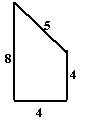The figure can be divided into a square and a right angle triangle.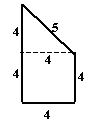Area = Area of square + Area of right angle triangle Area of the square = 4 x 4 = 16 cm2 Area of right angle triangle = 1/2 x 4 x 4 = 8cm2 Area of the figure = 16 + 8 = 24 cm2 Example 2: Find the area of the figure.The figure can be divided into 4 rectangles and 1 square.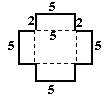Area = Area of 4 rectangles + Area of square Area of one rectangle = 5 x 2 = 10 cm2 Area of 4 rectangles = 4 x 10 = 40 cm2 Area of square = 5 x 5 = 25 cm2 Area = 40 + 25 = 65 cm2 Example 3: Find the area of the figure.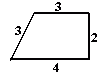The figure can be divided into parallelogram and a right angle triangle.Area = Area of parallelogram + Area of right angle triangle Area of parallelogram = 3 x 3 = 9 cm2 Area of right angle triangle = 1/2 x 1 x 2 = 1 cm2 Area = 9 + 1 = 10 cm2 Example 4: Find the area of the figure.The figure can be divided into two rectangles.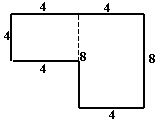Area = Area of square + Area of rectangle Area of square = 4 x 4 = 16 cm2 Area of rectangle = 8 x 4 = 32 cm2 Area = 16 + 32 = 48 cm2 Area = 9 + 1 = 10 cm2 Directions: Find the area of the figure.
 Q 1: Find the area of the figure.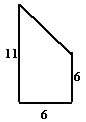Answer: Question 2: This question is available to subscribers only!

Subscription to kwizNET Learning System offers the following benefits:

• Unrestricted access to grade appropriate lessons, quizzes, & printable worksheets
• Instant scoring of online quizzes
• Progress tracking and award certificates to keep your student motivated
• Unlimited practice with auto-generated 'WIZ MATH' quizzes